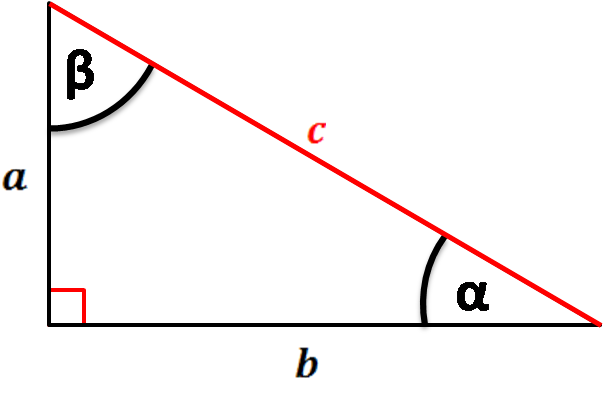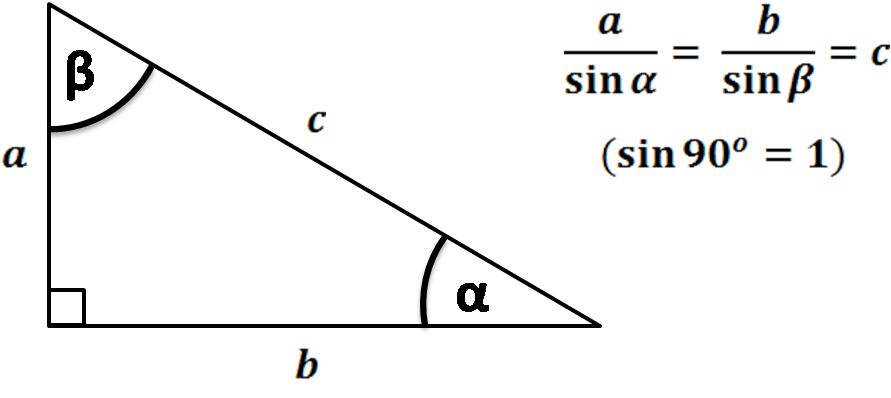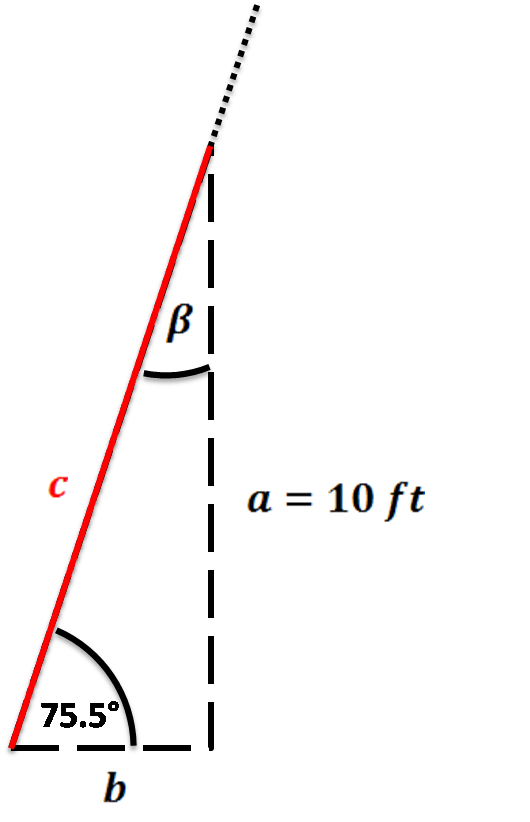# Hypotenuse Calculator

Created by Hanna Pamuła, PhD candidate
Reviewed by Bogna Szyk and Jack Bowater
Last updated: Aug 25, 2022

With this hypotenuse calculator you will quickly find this longest side of a right triangle. If you want to know what is the hypotenuse of a right triangle, how to find it and what is the hypotenuse of a triangle formula, you'll find the answer below, with a simple example to clear things up. Don't wait any longer, give this hypotenuse calculator a try!

## What is the hypotenuse of a triangleA hypotenuse is the longest side of a right triangle. It's the side that is opposite to the right angle (90°). Hypotenuse length may be found, for example, from the Pythagorean theorem.

## Hypotenuse of a triangle formulaThis hypotenuse calculator has a few formulas implemented - this way, we made sure it fits different scenarios you may encounter. You can find the hypotenuse:

1. Given two right triangle legs

Use the Pythagorean theorem to calculate the hypotenuse from right triangle sides. Take a square root of sum of squares:

• c = √(a² + b²)1. Given angle and one leg
• c = a / sin(α) = b / sin(β), from the law of sines
1. Given area and one leg

As area of a right triangle is equal to a * b / 2, then

• c = √(a² + b²) = √(a² + (area * 2 / a)²) = √((area * 2 / b)² + b²)

## How to find the hypotenuse of a right triangle with this hypotenuse calculator?Let's calculate how long the ladder should be if we want to rescue a kitten from a 10 ft roof. The calculator helps to find the ladder length from the ground to the edge of the roof, but don't forget about a ladder part which should extend over the edge!

1. Choose the option needed for your calculations. We know that our roof is 10 ft in height and that the safest angle for a ladder is ~75.5°. From the dropdown list of the given box, select the option: angle ∡ and one side.
2. Change the units to feet.1. Enter the given values. Our leg a is 10 ft long, and the α angle between ladder and ground equals 75.5°.

2. Ladder length, which is our right triangle hypotenuse, appears! It's equal to 10.33 ft.

The angle β = 14.5° and leg b = 2.586 ft are displayed as well. The second leg is also an important parameter, as it tells you how far the ladder should be removed from the wall (or rather from a roof edge). The general principle to remember is a 4:1 rule – for every four feet of vertical height, the ladder foot should move one foot from the wall.

## FAQ

### How do I find hypotenuse with sin?

1. Perform the sin operation on the angle (not the right angle).
2. Divide the length of the side opposite the angle from step 1 by the result of step 1.
3. The result is the hypotenuse.

### Is the hypotenuse always the longest side?

Yes, the hypotenuse is always the longest side, but only for right angled triangles. For isosceles triangles, the two equal sides are known as the legs, while in an equilateral triangle all sides are known simply as sides.

### How do I find the hypotenuse adjacent and opposite?

1. Find the longest side and label it the hypotenuse.
2. The adjacent and opposite can only be found if you choose one of the non right angled angles.
3. The adjacent is the side that forms the angle of choice along with the hypotenuse.
4. The opposite is the side that does not form the angle of choice.

### How do you find the altitude of a hypotenuse?

1. Draw the altitude of the hypotenuse on the triangle. The two new triangles you have created are similar to each other and the main triangle.
2. Divide the length of the shortest side of the main triangle by the hypotenuse of the main triangle.
3. Multiply the result by the length of the remaining side to get the length of the altitude.
4. Alternatively, the angles within the smaller triangles will be the same as the angles of the main one, so you can perform trigonometry to find it another way.

### What should I do if the hypotenuse is the opposite?

If the hypotenuse is the opposite, then you are considering the wrong angle - you cannot use trigonometry with right angle of a triangle. Consider one of the other angles, and the opposite will be the side that does not form that angle.

### How do I construct a perpendicular to the hypotenuse?

1. Acquire a pair of compasses, a ruler, and a pen or pencil.
2. Set your pair of compasses to the length of the hypotenuse (or any length, as long as it stays constant).
3. Draw a circle that has its center at one of the ends of the hypotenuse.
4. Draw another circle with its center at the other end of the hypotenuse.
5. Draw a line joining the two points where these circles meet. This line is the hypotenuse.

### How do I find the hypotenuse of isosceles right triangle?

1. Find the length of one of the non-hypotenuse sides.
2. Square the length of the side.
3. Double the result of the previous step.
4. Square root the result of step 3. This is the length of the hypotenuse.

### What is the hypotenuse angle theorem?

The hypotenuse angle theorem is a way of testing if two right angled triangles are congruent or not. It states that if two right angled triangles have a hypotenuse and an acute angle that are the same, they are congruent.

### Where does the word hypotenuse come from?

The word hypotenuse comes from the Ancient Greek hypoteinousa, meaning ‘stretching under (a right angle)’. This, in turn, comes from hypo- ‘under’, and teinein ‘to stretch’. Another thing we have to thank the Ancient Greeks for!

### How do I solve a right triangle with only the hypotenuse?

You cannot solve a right angled triangle with only the hypotenuse. This is because the other two sides and angles are still undefined and therefore the triangle can still have many forms.

Hanna Pamuła, PhD candidate
Given
two sides ∟a
in
b
in
Hypotenuse
c
in
People also viewed…

### Black Friday

How to get best deals on Black Friday? The struggle is real, let us help you with this Black Friday calculator!

### Car heat

The hot car calculator shows you how fast the interior of a car heats up during a summers day.

### Percentage of a percentage

Percentage of percentage allows you to multiply % by % and get the result.

### Perimeter of a triangle with fractions

Our perimeter of a triangle with fractions calculator will never give you mixed results even though you can input mixed numbers!